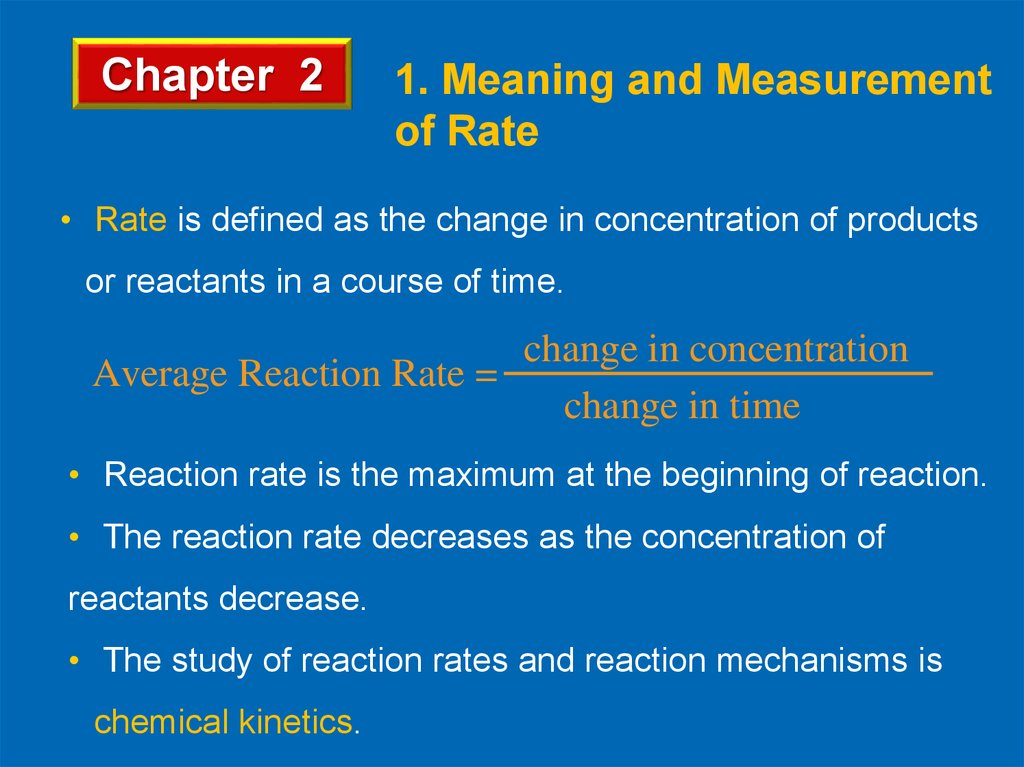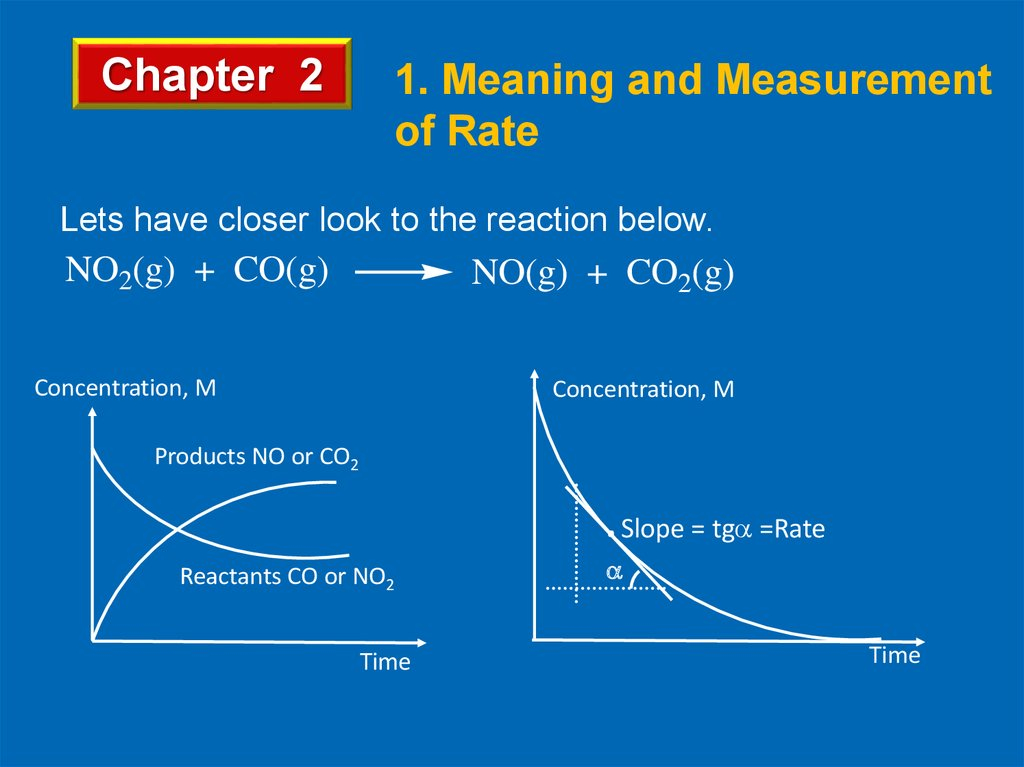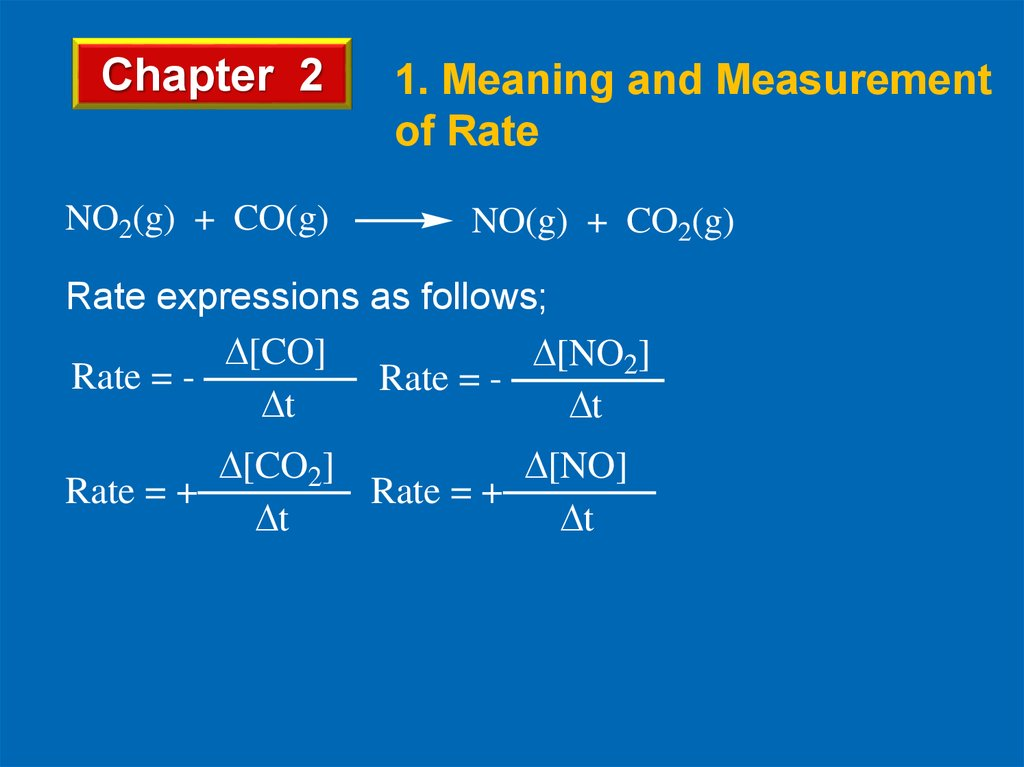Rate of reactions. (Chapter 2)

1. Slayt 1

Chapter 2
1. Meaning and Measurement
of Rate
• Rate is defined as the change in concentration of products
or reactants in a course of time.
change in concentration
Average Reaction Rate =
change in time
• Reaction rate is the maximum at the beginning of reaction.
• The reaction rate decreases as the concentration of
reactants decrease.
• The study of reaction rates and reaction mechanisms is
chemical kinetics.

2. Slayt 2

Chapter 2
1. Meaning and Measurement
of Rate
Lets have closer look to the reaction below.
NO2(g) + CO(g)
NO(g) + CO2(g)
Concentration, M
Concentration, M
Products NO or CO2
. Slope = tg =Rate
Reactants CO or NO2
Time
Time

3. Slayt 3

Chapter 2
NO2(g) + CO(g)
1. Meaning and Measurement
of Rate
NO(g) + CO2(g)
Rate expressions as follows;
[CO]
[NO2]
Rate = Rate = t
t
[CO2]
[NO]
Rate = +
Rate = +
t
t

4. Slayt 4

Chapter 2
1. Meaning and Measurement
of Rate
Example 1
Find the rate relationship of reactants and products for the
given reaction.
N2(g) + 3H2(g) 2NH3(g)
Solution
Rate expressions as follows;
[N2]
[H2]
Rate = Rate = t
t
Rate relationship
[N2]
[H2]
Rate = = t
t
[NH3]
Rate = +
t
[NH3]
=+
t

5. Slayt 5

• The decomposition of dinitrogen pentoxide
can be represented by the equation;
2N2O5 → 4NO2 + O2
• The concentration of dinitrogen pentoxide
decreases from 0,008 M to 0,004 M in 20
seconds. Find the average rate of consumption
of dinitrogen pentoxide

6. Slayt 6

• RateN2O5 = (0.008 – 0.004)/20 = 0.0002 =
2.10−4 mol/L. s

7. Slayt 7

Chapter 2
1. Meaning and Measurement
of Rate
Example 2
In the following decomposition reaction,
2N2O5 → 4NO2 + O2
oxygen gas is produced at the average rate of
9.1 × 10-4 mol · L-1· s-1. Over the same period, what is the
average rate of the following:
• the production of nitrogen dioxide.
• the loss of nitrogen pentoxide.

8. Slayt 8

Chapter 2
1. Meaning and Measurement
of Rate
Solution
rate NO2 production = 4 × (9.1 × 10-4 mol · L-1· s-1)
= 3.6 × 10-3 mol · L-1· s-1
rate loss of N2O5
= 2× (9.1 × 10-4 mol · L-1· s-1)
= 1.8 × 10-3 mol · L-1· s-1

9. Slayt 9

Chapter 2
3. Rate Expression and Rate
Constant
Consider the one step following reaction;
mA + nB → products
• The rate expression is proportional to the product of [A] (to
some power m) and [B] (to some power n). To create an
equation instead of a proportion, use the rate constant k.
Rate = k[A]m[B]n
• m and n are order of reaction with respect to related substance,
and k depends only on temperature and activation energy.
• Solids and liquids are not included in the expression, only
gaseous and aqueous ions are indicated in the rate expression.

10. Slayt 10

Chapter 2
3. Rate Expression and Rate
Constant
Example 10
Write the possible rate expression of the following reactions.
a. Cl2(g) + H2(g) → 2HCl(g)
b. Fe(s) + Cl2(g) → FeCl3(g)
c. Fe2O3 (s) + 3H2SO4 (aq) → Fe2(SO4)3 (s) + 3H2O(l)
d. Ca(s) + 2Ag+(aq) → 2Ca+2 (aq) + Ag(s)
Solution
a. Rate = k[Cl2][H2]
b. Rate = k[Cl2]
c. Rate = k[H2SO4]3
d. Rate = k[Ag+]2

11. Slayt 11

Chapter 2
3. Rate Expression and Rate
Constant
Example 11
Write the rate expression of the following one step reaction,
determine the order of reaction in terms of N2 and H2, and
overall order.
N2(g) + 3H2(g) → 2NH3(g)
Solution
Rate = k[N2][H2]3
Order in terms of N2 is 1 and 3 in terms of H2
Overall order is 1+3 = 4

12. Temperature

• The increase in temperature increases rate of
reaction. And rate can be calculated by the
formula below.
Vt2= Vt1. (t2 - t1)/10
is a constant
and given in the question.
Vt1 = velocity at initial temperature,
Vt2 = velocity at final temperature

13. Ex: If temperature increases 10 0C, rate increases 3 times. How many 0C must the temperature be increased so that rate can

increase 27 times?
If t2 - t1=10
Vt2 = 3Vt1
Then Vt2 = 3 = 10/10
3 = 1 so = 3
Vt1
If Vt2 = 27.Vt1
then
Vt2 = 27 = 3 (t2 - t1)/10
(t2 - t1)/ 10 = 3
Vt1
So t2 - t1 = 30 0C